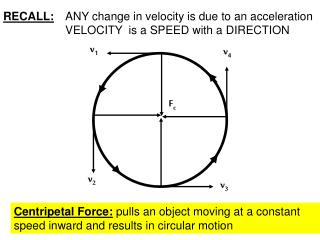DownloadDownload PresentationRECALL: ANY change in velocity is due to an acceleration VELOCITY is a SPEED with a DIRECTION

# RECALL: ANY change in velocity is due to an acceleration VELOCITY is a SPEED with a DIRECTION

Download Presentation## RECALL: ANY change in velocity is due to an acceleration VELOCITY is a SPEED with a DIRECTION

- - - - - - - - - - - - - - - - - - - - - - - - - - - E N D - - - - - - - - - - - - - - - - - - - - - - - - - - -
##### Presentation Transcript

1. v1 v4 v2 v3 RECALL: ANY change in velocity is due to an acceleration VELOCITY is a SPEED with a DIRECTION Fc Centripetal Force: pulls an object moving at a constant speed inward and results in circular motion

2. reaction action Newton’s Third Law of Motion To every action force there is an equal and opposite reaction force. While the forces are balanced, the motion that is observed depends upon the masses of the objects involved. (Rocket vs. exhaust gases)

3. Momentum: how much force is needed to change an object’s motion p = mv 1. What is the momentum of a car with a mass of 1300 kg traveling at a speed of 28 m/s? 2. What is the weight of the car in #1? p = mv = (1300 kg)(28 m/s) = 36,400 kg•m/s w = mg = (1300 kg)(9.8 m/s2) = 12,740 N

4. A few more examples: 1. A baseball thrown by a pitcher has a momentum of 6.0 kg•m/s. If the baseball’s mass is 0.15 kg, what is the baseball’s speed? 2. What is the mass of a person walking at a speed of 0.8 m/s if their momentum is 52.0 kg•m/s? 3. How fast must a 50 kg mass travel to have a momentum of 1500 kg •m/s? 4. If a 5000 kg mass is moving at a speed of 43 m/s, what is its momentum? p = mv v = 400 m/s 6.0 kg•m/s = (0.15 kg)v p = mv 52.0 kg•m/s = m(0.8 m/s) m = 65 kg v = 300 m/s p = mv 1500 kg•m/s = (50 kg)v p = mv = (5000 kg)(43 m/s) = 21,500 kg•m/s

5. Law of Conservation of Momentum: Momentum can be transferred from one object to another. In a closed system, there is no gain nor loss of the total momentum of all of the objects.